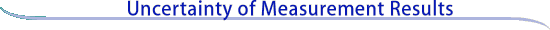Examples of uncertainty statements

The following are examples of uncertainty statements as would be used in publication or correspondence. In each case, the quantity whose value is being reported is assumed to be a nominal 100 g standard of mass ms.

Example 1
ms = 100.021 47 g with a combined standard uncertainty (i.e., estimated standard deviation) of uc = 0.35 mg. Since it can be assumed that the possible estimated values of the standard are approximately normally distributed with approximate standard deviation uc, the unknown value of the standard is believed to lie in the interval ms ± uc with a level of confidence of approximately 68 %.

Example 2
ms = (100.021 47 ± 0.000 70) g, where the number following the symbol ± is the numerical value of an expanded uncertainty U = k uc, with U determined from a combined standard uncertainty (i.e., estimated standard deviation) uc = 0.35 mg and a coverage factor k = 2. Since it can be assumed that the possible estimated values of the standard are approximately normally distributed with approximate standard deviation uc, the unknown value of the standard is believed to lie in the interval defined by U with a level of confidence of approximately 95 %.

Continue to Background